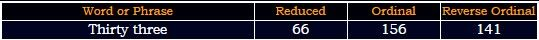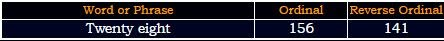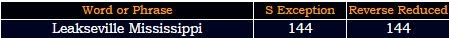## Cipher## Mar 23, 2017

### Bill Hicks = Alex Jones - Beautiful gematria script

There's a lot of people showing evidence that Bill Hicks is Alex Jones but when we look at gematria we see insane relationship between these two charactersBill Hicks "died" on 2/26/1994 - 2+2+6+1+9+9+4 = 33 - he was born in "Valdosta Georgia" = 4+1+3+4+6+1+2+1 + 7+5+6+9+7+9+1 = 66 (Reduced)
"Valdosta Georgia" = 22+1+12+4+15+19+20+1 + 7+5+15+18+7+9+1 = 156 (Ordinal)2/26/1994 - 2+26+19+94 = 141
He's born on 16th day of the December - Sixteen = 1+9+6+2+5+5+5 = 33 (Reduced)

Note that Alex Jones is supposedly born in "Dallas, Texas" = 4+1+3+3+1+1 + 2+5+6+1+1 = 28 (Reduced)
Hicks was born on 12/16 = 28 and he died on 2/26 which also sums to 28..156th prime number is 911
Note that Alex Jones is probably most known for his 9/11 stuff and Bill Hicks died EXACTLY 1 year after 1993 World Trade Center Bombing
"one year" = 60+50+5 + 700+5+1+90 = 911 (English)
Bill Hicks is listed as a musician and comedian, he "died" at Little Rock which connects to his profession
"Little Rock" = 3+9+2+2+3+5 + 9+6+3+2 = 44 (Reduced)
comedian = 6+3+5+4+5+9+8+4 = 44 (Reverse Reduced)
musician = 4+3+10+9+3+9+1+5 = 44 (S Exception)
He was also born in "December" = 5+4+6+4+5+7+4+9 = 44 (Reverse Reduced)
He supposedly died from "Pancreatic cancer"
Pancreatic cancer = 7+1+5+3+9+5+1+2+9+3 + 3+1+5+3+5+9 = 71 (Reduced)
cancer = 3+1+14+3+5+18 = 44 (Ordinal)Magnolia = 14+26+20+13+12+15+18+26 = 144 (Reverse Ordinal)Hicks was born in "Georgia" = 7+5+6+9+7+9+1 = 44 (Reduced)

Now here is the best part
"Bill Hicks" = 2+9+30+30 + 8+9+3+20+100 = 211 (English)
He died 2 months and 11 days after his birthdayAlex Jones is born on 2/11 - the 42nd day of the year
"February" = 6+5+2+9+3+1+9+7 = 42 (Reduced)
Forty second day = 6+6+9+2+7 + 1+5+3+6+5+4 + 4+1+7 = 66 (Reduced)
Alex Jones = 1+3+5+6 + 1+6+5+5+1 = 33 (Reduced)
Alex Jones = 1+3+5+6 + 1+6+5+5+10 = 42 (S Exception)
InfoWars = 9+5+6+6+5+1+9+1 = 42 (Reduced)

His last comedy special is called Revelations and it was aired on September 14th, 93 days before his birthdayHis birthday in 1993 landed on a date with 32 numerology (12/16/1993 - 1+2+1+6+1+9+9+3 = 32)
Bill Hicks died at the age of 32 - "Hicks" = 8+9+3+2+10 = 32 (S Exception)
World Trade Center bombing took place on  2/26/93 "Two hundred twenty six" = 2+5+6 + 8+3+5+4+9+5+4 + 2+5+5+5+2+7 + 1+9+6 = 93 (Reduced)

Check out this video, this guy shows pretty nice proof of them being the same person
1.1.